# Level Sets of the Value Function in Linear Differential Games with Elliptical Vectograms

## Title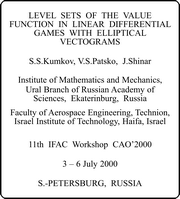Our paper is a collection of four examples from the differential game theory. We consider linear differential games with fixed terminal time. For such games, a level set of the value function is a tube in the game space "phase vector × time". If a game is regular, then every level set tube is smooth. Non-smoothness and some other geometrical features of the tubes can tell us about difficulties of the solution of the game.

## Slide 1

Let us imagine a problem where a rocket pursues another one. At the beginning, their nominal velocities and courses are such that a collision is forecasted. The velocities are quite large (and the nominal relative velocity is very large also). The pursuer and evader controls are not too strong to change the trajectories sufficiently. They can only correct the trajectories slightly. The pursuer minimizes the miss distance, the evader maximizes it. It is reasonable to measure the miss distance not as the shortest distance between the objects during the homing process, but as the distance between projections of the geometrical states of the objects at the instant of the nominal collision into the plane orthogonal to the direction of the nominal relative velocity. Let take axes in this plane as follows: the first axis is defined by the vectors of the nominal velocities (that is the first axis is situated in the plane of the nominal velocities vectors), and the second one is orthogonal to it.

We consider the game from the point of view of the first player (the pursuer). So, one can take into account the inertia of the servo-mechanisms of the pursuer in the statement of the problem. About the second player (the evader) it is supposed that its control signals are realized instantly.

It is natural to linearize the dynamics of the objects along the nominal motions.

Thus, we have a linear differential game with fixed terminal time.

## Slide 2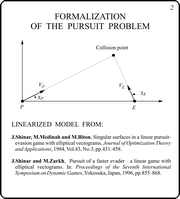An investigation of such linearized problem was made in the works of J.Shinar and his collaborators. In the Figure, the nominal motions and the forecasted collision point are shown. It is supposed that the accelerations of both vehicles are bounded and orthogonal to the respective nominal velocity vectors. The corresponding dynamics vectograms are circular.

## Slide 3

We consider planar motions in the plane orthogonal to the initial nominal relative velocity. When projecting the original circular control constraints into this plane, the new constraints become elliptical.

In the slide, the linearized dynamics is shown. The phase vector is six-dimensional (two geometrical difference coordinates, two difference velocities, two difference accelerations). The constant kP describes the inertia of servo-mechanisms of the pursuer. The miss is computed as the distance from the origin to the difference geometrical state at the terminal time.

In the papers of J.Shinar and his collaborators, the results of analytical investigations of this linear differential game were presented. The relations describing the level sets of the value function were obtained. It was established that the value function is not differentiable. Singular surfaces were analyzed. The system of singular surfaces for this problem contains dispersal, equivocal and focal pieces.

All computations were made by means of the equivalent coordinates of the dimension two. The transformation from the original six-dimensional vector to the equivalent one is provided by the standard variable change which involves the fundamental Cauchy matrix for the system dz/dt = A(t)z (without the controls). The game space is three-dimensional: two equivalent coordinates and the time.

## Slide 4

After the transformation to the equivalent coordinates, the dynamics of the game has the form shown in the slide. The terminal instant and control constraints are the same as in the original game. The payoff function is the norm of the equivalent phase vector x at the terminal instant.

Further, some results numerically obtained for this game are presented. Standard programs for solving differential games developed in the Institute of Mathematics and Mechanics (Ekaterinburg, Russia) were used. Numerical data for the example are shown in the slide.

## Slide 5

To understand the structure of level sets of the value function, it is useful to consider tubes of players vectograms. The tube of vectograms of the first player is the set P(t)=X1,4(T,t)BP enrolled in time. In the same way, the tube of vectograms of the second player is understood.

In the Figure, the first player's tube of vectograms is shown by red color, and the second player's one is green. The latter is made transparent. Contours of some t-sections are drawn on it. The symbol t denotes the backward time.

In this example, the second player's tube of vectograms is an elliptical cone. The first player's tube also has elliptical t-sections which grow non-linearly. For small values of the backward time t, the second player has full advantage: t-sections of his tube include corresponding t-sections of the tube of the second player. After some instant, the first player gets advantage in vertical direction and in directions close to the vertical one. With increasing the backward time, the first player takes the full advantage.

## Slide 6In this slide, a level set of the value function is shown. It corresponds to a value c* of the payoff function. This level set is infinite in time. It has a narrow throat. After the throat, the level set enlarges with growing the backward time.

The considered value c* is the critical one: for less values of c, corresponding level sets are finite in time. With small decreasing of the parameter c, level set breaks near the throat.

In the picture, the changing of t-section orientation can be seen: before the throat they are oriented horizontally, after the throat they become oriented vertically. Namely, these facts give difficulties to the solution of the considered differential game. In this situation, the numerical results show adequately the structure of level sets near the throat and correspond well to the analytical results only if very precise computations are used.

## Slide 7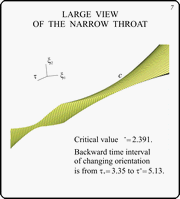This slide contains a zoomed view of the narrow throat. Contours of some t-sections are shown.

## Slides 8, 9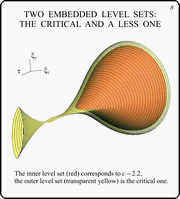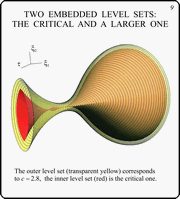These two slides show the critical level set with close ones. The slide 8 contains the critical level set (yellow transparent) and a set corresponding to a bit less value of the parameter c (red). The slide 9 demonstrates the critical level set (red) with a set corresponding to a bit greater value of the parameter c (yellow transparent).

In the theory of differential games, there is a well-known example "the boy and the crocodile". This game has also a narrow throat for critical tube. But t-sections are circular in the game "the boy and the crocodile". The J.Shinar's example is more complicated.

The following question is very natural: how typical is the situation of presence of throats, and how many throats can a level set have?

With this object in mind, the authors have turned to the class of differential games known in Russian literature on differential games as the "L.S.Pontryagin's generalized test example".

## Slide 10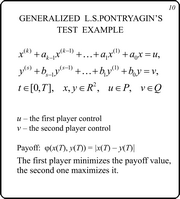The slide shows the dynamics of the L.S.Pontryagin's generalized test example. The case is considered when the objects move in a plane, that is the x and y are two-dimensional vectors. The terminal time of the game is fixed. The payoff function is defined as the geometrical distance between the objects at the terminal instant. The first player minimizes the payoff function, the second one maximizes it.

## Slide 11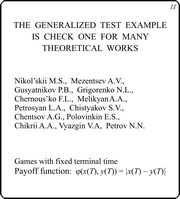Here is a list of authors who investigated the L.S.Pontryagin's generalized test example or used it as a check one for new theoretical methods. Very often, the time of termination is not fixed. The game with fixed terminal time appears in this case as a basis for the solution.

As a rule, the constraints P and Q were taken circular. We consider the case when the constraints P and Q are ellipses.

## Slide 12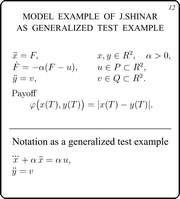In the model example investigated by J.Shinar, the evader governs a material point with inertialess control, and the pursuer has inertial control circuit. The dynamics can be rewritten as it is shown at the bottom of the slide. So, this problem is a particular case of the L.S.Pontryagin's test example.

## Slide 13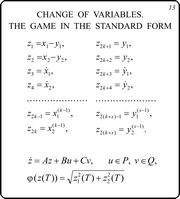With introducing new variables, the dynamics of the test example can be presented in the standard form.

## Slide 14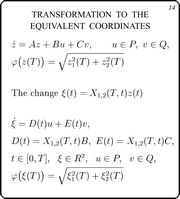The payoff function depends only on two components of the phase vector at the terminal instant. So, by means of the well-known change of variables which involves two rows of the fundamental Cauchy matrix, one can pass to the equivalent game. The phase vector of the equivalent game is two-dimensional.

Three following slides describe shortly the idea of the backward computational procedure for constructing a collection of t-sections of a level set of the value function.

## Slide 15This slide explains the scheme of the backward procedure. The algorithm starts with the payoff function level set (Lebesgue set) corresponding to a number c. Then a collection of t-sections of the value function level set is constructed for a given time grid. This level set corresponds to the chosen number c. In general, this is the method of dynamical programming in application to differential games.

## Slide 16For numerical procedure, some approximations are made. The dynamics of the equivalent games is changed by a piecewise-constant one. The constraints P and Q are substituted by convex polygons. The payoff function j is approximated by a function which has convex polygonal level sets.

## Slide 17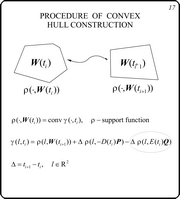The transformation from one section to the next one is provided by a specialized procedure. In its basis, it has an algorithm for constructing convex hull of a positively homogenious piecewise-linear function. The formula for computation of this function is shown in the slide. Here, D is the step of the time grid.

Further, three example of differential games are presented. Some their level sets have narrow throats. It was not too easy to find these examples. But authors are sure that the presence of such throats is quite typical.

## Slide 18The dynamics of the first example is written in this slide. The first player governs an inertial point in the plane. The second object is a two-dimensional mathematical pendulum. Both objects have friction proportional to the velocity. The constraints on the player controls are taken as ellipses.

## Slide 19

In this slide, two views of the tubes of vectograms for both players are presented. The green tube belongs to the first player, the red one is of the second.

Since the second player dynamics is oscillating, the advantage changes in time. At the beginning of the backward time, the second player has full advantage, but for sufficiently large values of the backward time t, the first player gets the advantage.

## Slide 20

This slide shows the level set for c = 2.451. One can see the varying orientation of t-sections of the set. At the beginning, they are have vertically elongated orientation. Further, t-sections become horizontally elongated. This change is determined by delicate interaction of the vectograms.

This example was computed in the interval t Î [0,20]. The time step D = 0.05. The level set of the payoff function j was 100-gon. The ellipses P and Q were approximated by polygons with 100 vertices.

## Slide 21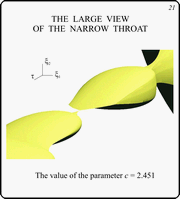In this picture, a zoomed view of the tube is drawn. It can be seen that the level set degenerates almost to a point.

## Slide 22

Here, the second example is presented. In this case, both players govern oscillating objects. It is noticeable that the constraints on the player controls are taken as coinciding ellipses.

Despite the ellipses of the constraints coincide, the computations are not trivial, because there is no uniformity of the players vectograms and level sets of the payoff function.

## Slides 23,24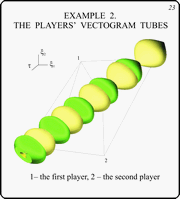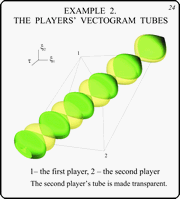These slides demonstrate the tubes of vectograms for the second example. The green tube belongs to the first player, the yellow one is of the second. In the 24th slide, the latter is transparent.

## Slide 25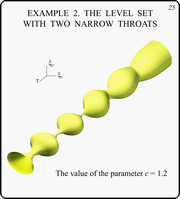This slide gives the general view of the level set for c = 1.2. This level set has two narrow throats. They correspond to the instants t1 = 5.65 and t2 = 8.50.

This example was computed in the interval
t Î [0,20]. The time step D = 0.05. Near the throats, 10 times less step was used: D = 0.005. The level set of the payoff function j was approximated by a regular 100-gon. The ellipses P and Q were approximated by polygons with 100 vertices.

## Slide 26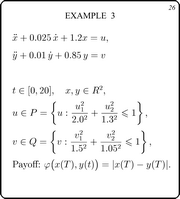Here, the dynamics of the third example is shown. Again, two oscillators with elliptical constraints.

## Slide 27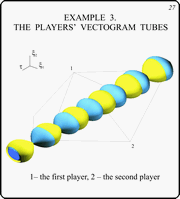In this figure, the tubes of the players vectograms are drawn.

## Slide 28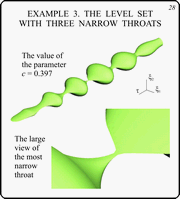In this slide, the level set having three narrow throats is demonstrated. The level set corresponds to c = 0.397.

## Slide 29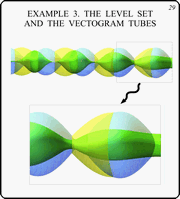Here, the tubes of vectograms are put on the level set. All colors correspond to ones used before: the blue tube is the tube of the first player vectograms, the yellow tube is of the second player vectograms, and the green one is the level set. The tubes of vectograms are transparent.

Variation of the level set in time is clearly connected to the character of this or that player advantage. When the second player has full advantage (when the yellow tube contains the blue one completely), the level set contracts. Vice versa, when the first player gets full advantage, the level set enlarges. If no player has full advantage (that is both players have partial advantage), the situation with the level set changing can be more complicated: growth to some directions and decreasing to others could take place.

## The Whole Booklet (all slides and comments in PDF)

S.S. Kumkov, V.S. Patsko
Institute of Mathematics & Mechanics Ural Branch of RAS
S.Kovalevskaya str.16
620219 Ekaterinburg, Russia
e-mail:
sskumk@gmail.com
patsko@imm.uran.ru

J.Shinar
Faculty of Aerospace Engineering, Technion - Israel Institution of Technology,
Haifa, 32000, Israel,
e-mail:
aer4301@aerodyne.technion.ac.il